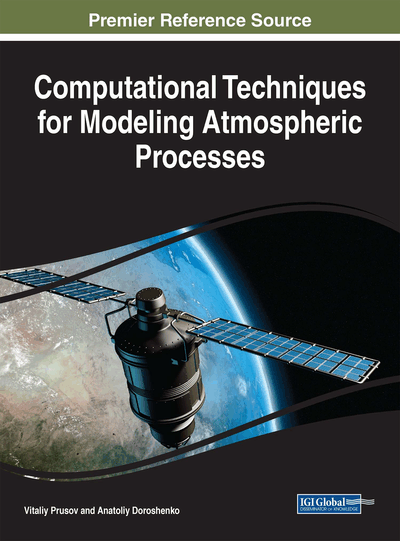# Equations Satisfied Physical Processes in the Atmosphere

DOI: 10.4018/978-1-5225-2636-0.ch003
Available
\$37.50
No Current Special Offers

## Abstract

The chapter considers theoretical foundation of the modern hydro-mechanics in their application to the atmosphere dynamics problems. General concepts, definitions, and notation needed for mathematical modeling atmospheric processes, particularly, the elements of kinematics and deformation of continuous media, and curved orthogonal coordinates are introduced. The basic equations of mechanics of viscous stratified fluid are considered including the equations expressing the law of conservation of mass, the equations expressing the law of momentum, the equations expressing the law of conservation of energy, the concentration equations for mechanics of heterogeneous medium. At the end of the chapter, the complete list of the basic equations of the model of atmosphere is given and the initial and boundary conditions in the hydrodynamic problems are described.
Chapter Preview
Top

## Preliminary Notes

Cognition of nature and solving many related urgent tasks require the construction of new and improvement of existing models for deeper and more detailed description of the microscopic and macroscopic physical objects, interactions, and events. The experience and the inherent essence of science indicate that the answers to the questions of meteorology, to various questions about the material properties of the complicated interactions of the inorganic world is largely due to some of our common universal concepts, ideas, laws, and methods.

To date, it has already accumulated huge scientific information, developed the theory and collected observations data on the behavior of meteorological fields on the flow and balance of the atmosphere, homogeneous air masses and heterogeneous formations.

Explicit establishment of common principles and internal connections between different theories and observed effects contribute to a deeper understanding of the actual state of science, the correct assessment of the known and emerging scientific achievements and best orientation in the wealth extracted information. These can serve as a starting basis for further scientific development.

In presenting the theoretical foundations of fluid mechanics and thermodynamics it is useful to be guided by the following provision, which must be emphasized clearly. Introduced and accepted concepts and their relations have some precise meaning only within the framework of a set of models that are designed for a scientific description of the domain of real phenomena that we are interested in. This also applies to such fundamental concepts as space, time, power, speed, temperature, entropy, etc. As we know, the idea of a Newtonian force is totally inadequate to describe the mechanical interactions in the modern sophisticated models of continuum mechanics.

Therefore, it is reasonable to talk about the basic concepts and laws in the framework of the aggregate of wide or narrow classes of models that have already been introduced explicitly or may be introduced. The necessary clarity is achieved by determining the correct models where the emerging contradictions can be easy to handle. But, obviously, that all the models reflect reality only approximately and only in a certain region. Refinement, sophistication, a new simulation or in a way simplification of the existing models – is an ongoing process related to scientific progress.

Theoretical foundations of dynamic meteorology are particularly useful not only in terms of the already known applications but in terms of future challenges, which will be the subject of research and applications in the future. In this regard, in recent years it has clearly revealed the need to address in dynamic meteorology as a common basis for the development of thermodynamics, hydrodynamics, circulation of the atmosphere, physical processes of evaporation and condensation of steam. Commonality and the inextricable relation of these sections of meteorology and physics force us to consider them as a whole.

The modern theory of nonlinear continuum mechanics based on approximations of a Newtonian fluid allows representing a highly complex subject of dynamic meteorology in the form of a system of equations that reflect the corresponding physical laws and are well proven in many applied problems of hydrodynamics.

In general, these equations include following:

• Thermodynamic equation.

• Mass balance equations.

• Momentum balance equation.

• Rheological equation.

• The concentration balance equation.

• The energy balance equation.

• The equation of state, which connects the internal energy with temperature, density and the strained state of the medium.

• Fourier (Fick) law, which establishes the proportional relationship between the heat flux (mass) and temperature gradient (concentration).

## Complete Chapter List

Search this Book:
Reset